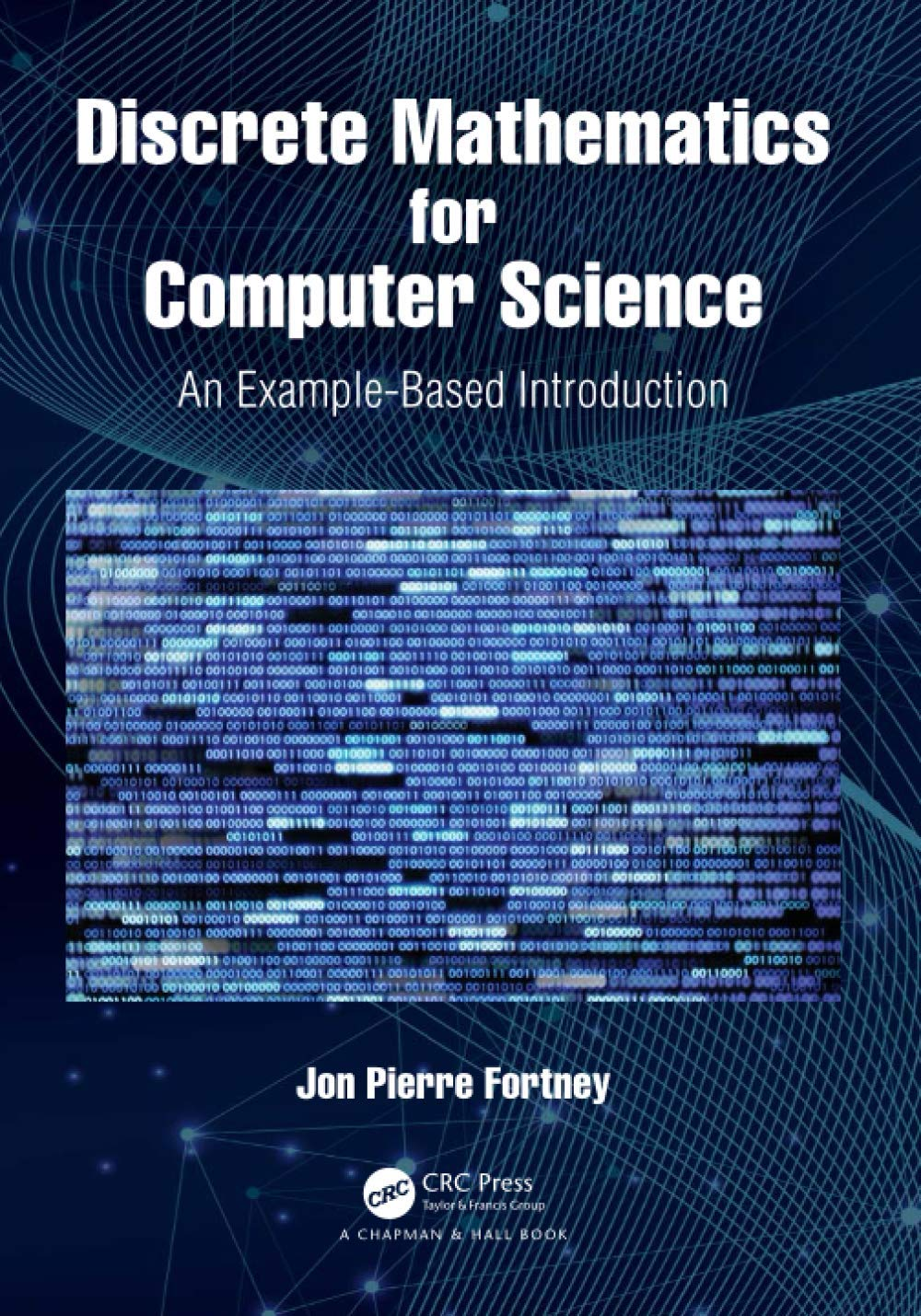# Discrete Mathematics for Computer Science: An Example-Based IntroductionPrice: ₹5,136.00
(as of Oct 23,2021 18:29:13 UTC – Details)Discrete Mathematics for Computer Science: An Example-Based Introduction is intended for a first- or second-year discrete mathematics course for computer science majors. It covers many important mathematical topics essential for future computer science majors, such as algorithms, number representations, logic, set theory, Boolean algebra, functions, combinatorics, algorithmic complexity, graphs, and trees.

Features

• Designed to be especially useful for courses at the community-college level
• Ideal as a first- or second-year textbook for computer science majors, or as a general introduction to discrete mathematics
• Written to be accessible to those with a limited mathematics background, and to aid with the transition to abstract thinking
• Filled with over 200 worked examples, boxed for easy reference, and over 200 practice problems with answers
• Contains approximately 40 simple algorithms to aid students in becoming proficient with algorithm control structures and pseudocode
• Includes an appendix on basic circuit design which provides a real-world motivational example for computer science majors by drawing on multiple topics covered in the book to design a circuit that adds two eight-digit binary numbers

Jon Pierre Fortney graduated from the University of Pennsylvania in 1996 with a BA in Mathematics and Actuarial Science and a BSE in Chemical Engineering. Prior to returning to graduate school, he worked as both an environmental engineer and as an actuarial analyst. He graduated from Arizona State University in 2008 with a PhD in Mathematics, specializing in Geometric Mechanics. Since 2012, he has worked at Zayed University in Dubai. This is his second mathematics textbook.

Scroll to Top